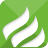# bignumber.js，javascript前端高精度计算库推荐

BigNumber.js 是一个用于 JavaScript 的库，它提供了高精度数学运算功能，可以解决 JavaScript 浮点数精度问题。这个库允许你在 JavaScript 中执行精确的大数运算，而不会丢失精度或遇到浮点数舍入误差。

``````npm install bignumber.js
``````

``````yarn add bignumber.js
``````

``````const BigNumber = require('bignumber.js');
``````

``````// 加
let c = new BigNumber(a).plus(b).toNumber();

// 减
let d = new BigNumber(a).minus(b).toNumber();

// 乘
let e = new BigNumber(a).multipliedBy(b).toNumber();

// 除
let f = new BigNumber(a).dividedBy(b).toNumber();
``````

// 更多运算和功能请参考 BigNumber.js 文档BigNumber.js 这个库的官方文档提供了更详细的信息和示例，以帮助你更好地了解如何使用它：
https://mikemcl.github.io/bignumber.js/

PS：bignumber.js的特点就是处理整数和小数，跟decimal.js是一个作者，具体也可以了解一下decimal.js。

0.101768s